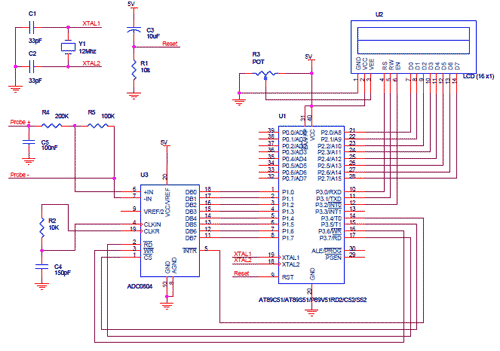# Methods for measuring power consumed by a ciruit

1. ## Methods for measuring power consumed by a ciruit

What are the methods for measuring the current consumed by a circuit and out put in a LCD2. ## Re: Methods for measuring power consumed by a ciruit

What is the current range?
1. measure the voltage drop across a resistor in series with the load - simple ohms law, a 1 ohm resistor will drop 1V for every amp of current. it's usual to use a smaller resistor and amplify the voltage drop with an opamp - this also allows you to reference the voltage to 0V (by using a differential opamp circuit).

Use ADC to convert -> microcontroller->output to LCD
For idea:----------- Post added at 00:51 ---------- Previous post was at 00:44 ----------

1 members found this post helpful.•

3. ## Re: Methods for measuring power consumed by a ciruit

inside a 10 x 7W LED4. ## Re: Methods for measuring power consumed by a ciruit

LCD's use tiny amounts of current, compared to LED's.

See if you can spot any variation in the overall current draw when the display shows all 8's (all segments lit up), as compared to when few segments are lit up. That might give you a way to estimate what's going on in terms of power consumption.•

5. ## Re: Methods for measuring power consumed by a ciruit

You can always measure the current at source (for total current withdrawn) or consumed at load (total current consumed), Your question is not very clear, do you mean methods to measure the current?6. ## Re: Methods for measuring power consumed by a ciruit

My intention is to measure the current consumed by a series of LEDs ,
To measure the power by normal mode and that saved by PWM mode by these in series7. ## Re: Methods for measuring power consumed by a ciruit

I think you'll need to use this method:

Measure the voltage across your switching device while it's conducting.

Also measure current through the switching device while it's conducting. This may not be easy. If you have an oscilloscope then put a low-ohm resistor inline and measure voltage across it.

Multiply V * A.

This will give you instantaneous watts wasted as heat through the device.

Multiply by your duty cycle. This will give you average watts wasted as heat.

Now you will calculate the theoretical waste if you used a linear resistive method to drop voltage.

You know the average applied voltage on the LED's, and the average current going through them. You also know your supply voltage. From these figures you can calculate what amount of power you would have been wasting.8. ## Re: Methods for measuring power consumed by a ciruitOriginally Posted by papunblgWhat is the current range?
1. measure the voltage drop across a resistor in series with the load - simple ohms law, a 1 ohm resistor will drop 1V for every amp of current. it's usual to use a smaller resistor and amplify the voltage drop with an opamp - this also allows you to reference the voltage to 0V (by using a differential opamp circuit).

Use ADC to convert -> microcontroller->output to LCD
For idea:----------- Post added at 00:51 ---------- Previous post was at 00:44 ----------

I agree with your post sir,
:D
I want to ask how to make characters at row 2 in LCD sir? how its program?
visit my site also ELECTRONICS IS FUN9. ## Re: Methods for measuring power consumed by a ciruit

I dont want accuracy in the values we get, I want to calculate just the rough value in power we saved.
Btw, any PIC based project available for this ?•

10. ## Re: Methods for measuring power consumed by a ciruit

Pic16F876A(for example) contains inbuilt ADC. So the circuit will be simple as there is no external ADC in this case. Search this forum.--[[ ]]--